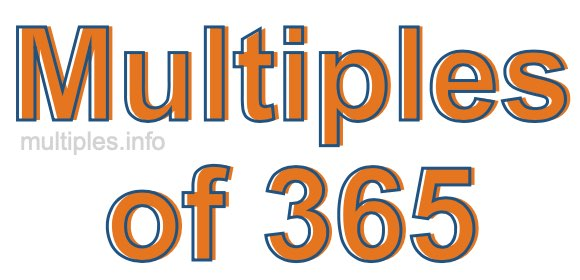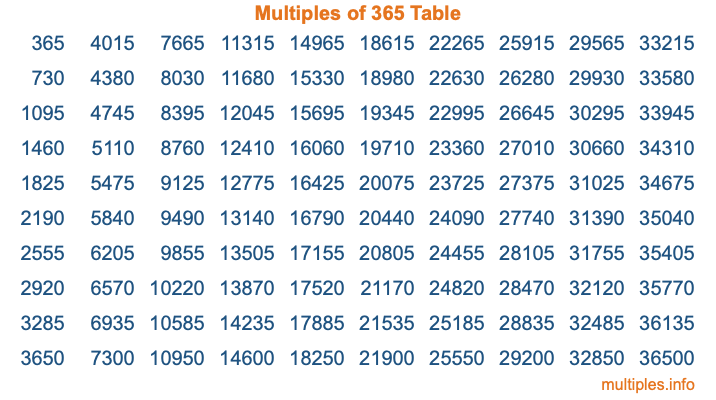Multiples of 365Welcome to the Multiples of 365 page. Here we will first teach you everything you will ever need to know about the multiples of 365, and then give you a study guide summary of everything we taught you to make sure you remember it all. Use this page to look up facts and learn information about the multiples of 365. This page will make you a multiples of three hundred sixty-five expert!

Definition of Multiples of 365
Multiples of 365 are all the numbers that when divided by 365 equal an integer. Each of the multiples of 365 are called a multiple. A multiple of 365 is created by multiplying 365 by an integer.

Therefore, to create a list of multiples of 365, you start with 1 multiplied by 365, then 2 multiplied by 365, then 3 multiplied by 365, and so on for as long as you want. Thus, the list of the first five multiples of 365 is 365, 730, 1095, 1460, and 1825. To see a larger list of multiples of 365, see the printable image of Multiples of 365 further down on this page. We also have a category where you can choose any nth multiple of 365.

Multiples of 365 Checker
The Multiples of 365 Checker below checks to see if any number of your choice is a multiple of 365. In other words, it checks to see if there is any number (integer) that when multiplied by 365 will equal your number. To do that, we divide your number by 365. If the the quotient is an integer, then your number is a multiple of 365.

Is  a multiple of 365?

Least Common Multiple of 365 and ...
A Least Common Multiple (LCM) is the lowest multiple that two or more numbers have in common. This is also called the smallest common multiple or lowest common multiple and is useful to know when you are adding our subtracting fractions. Enter one or more numbers below (365 is already entered) to find the LCM.

Check out our LCM Calculator if you need more details about the Least Common Multiple or if you need the LCM for different numbers for adding and subtraction fractions.

nth Multiple of 365
As we stated above, 365 is the first multiple of 365, 730 is the second multiple of 365, 1095 is the third multiple of 365, and so on. Enter a number below to find the nth multiple of 365.

th multiple of 365

Multiples of 365 vs Factors of 365
365 is a multiple of 365 and a factor of 365, but that is where the similarities end. All postive multiples of 365 are 365 or greater than 365. All positive factors of 365 are 365 or less than 365.

Below is the beginning list of multiples of 365 and the factors of 365 so you can compare:

Multiples of 365: 365, 730, 1095, 1460, 1825, etc.

Factors of 365: 1, 5, 73, 365

As you can see, the multiples of 365 are all the numbers that you can divide by 365 to get a whole number. The factors of 365, on the other hand, are all the whole numbers that you can multiply by another whole number to get 365.

It's also interesting to note that if a number (x) is a factor of 365, then 365 will also be a multiple of that number (x).

Multiples of 365 vs Divisors of 365
The divisors of 365 are all the integers that 365 can be divided by evenly. Below is a list of the divisors of 365.

Divisors of 365: 1, 5, 73, 365

The interesting thing to note here is that if you take any multiple of 365 and divide it by a divisor of 365, you will see that the quotient is an integer.

Multiples of 365 Table
Below is an image of the first 100 multiples of 365 in a table. The table is in chronological order, column by column. The first column has the first ten multiples of 365, the second column has the next ten multiples of 365, and so on.The Multiples of 365 Table is also referred to as the 365 Times Table or Times Table of 365. You are welcome to print out our table for your studies.

Negative Multiples of 365
Although not often discussed or needed in math, it is worth mentioning that you can make a list of negative multiples of 365 by multiplying 365 by -1, then by -2, then by -3, and so on, to get the following list of negative multiples of 365:

-365, -730, -1095, -1460, -1825, etc.

Multiples of 365 Summary
Below is a summary of important Multiples of 365 facts that we have discussed on this page. To retain the knowledge on this page, we recommend that you read through the summary and explain to yourself or a study partner why they hold true.

There are an infinite number of multiples of 365.

A multiple of 365 divided by 365 will equal a whole number.

365 divided by a factor of 365 equals a divisor of 365.

The nth multiple of 365 is n times 365.

The largest factor of 365 is equal to the first positive multiple of 365.

365 is a multiple of every factor of 365.

365 is a multiple of 365.

A multiple of 365 divided by a divisor of 365 equals an integer.

365 divided by a divisor of 365 equals a factor of 365.

Any integer times 365 will equal a multiple of 365.

Multiples of a Number
Here you can get the multiples of another number, all with the same attention to detail as we did for multiples of 365 on this page.

Multiples of
Multiples of 366
Did you find our page about multiples of three hundred sixty-five educational? Do you want more knowledge? Check out the multiples of the next number on our list!